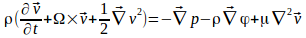## Equation of Motion For a Viscous Fluid

The eqution of motion for an inviscid fluid is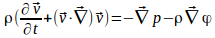< >where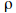is the density,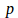is the pressure and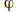is the potential as a function of position.

< >To include viscosity effects we can just add a viscosity term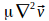to the right hand side.

< >The equation becomes

< >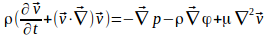< >Use the identity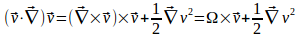< >where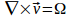is the vorticity.

< >